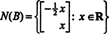## The Nullspace of a Matrix

The solution sets of homogeneous linear systems provide an important source of vector spaces. Let A be an m by n matrix, and consider the homogeneous system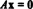Since A is m by n, the set of all vectors x which satisfy this equation forms a subset of R n . (This subset is nonempty, since it clearly contains the zero vector: x = 0 always satisfies A x = 0.) This subset actually forms a subspace of R n , called the nullspace of the matrix A and denoted N(A). To prove that N(A) is a subspace of R n , closure under both addition and scalar multiplication must be established. If x 1 and x 2 are in N(A), then, by definition, A x 1 = 0 and A x 2 = 0. Adding these equations yieldswhich verifies closure under addition. Next, if x is in N(A), then A x = 0, so if k is any scalar,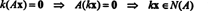verifying closure under scalar multiplication. Thus, the solution set of a homogeneous linear system forms a vector space. Note carefully that if the system is not homogeneous, then the set of solutions is not a vector space since the set will not contain the zero vector.

Example 1: The plane P in Example 7, given by 2 x + y − 3 z = 0, was shown to be a subspace of R 3. Another proof that this defines a subspace of R 3 follows from the observation that 2 x + y − 3 z = 0 is equivalent to the homogeneous system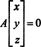where A is the 1 x 3 matrix [2 1 −3]. P is the nullspace of A.

Example 2: The set of solutions of the homogeneous systemforms a subspace of Rn for some n. State the value of n and explicitly determine this subspace.

Since the coefficient matrix is 2 by 4, x must be a 4‐vector. Thus, n = 4: The nullspace of this matrix is a subspace of R4. To determine this subspace, the equation is solved by first row‐reducing the given matrix:Therefore, the system is equivalent to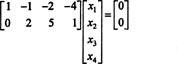that is,If you let x 3 and x 4 be free variables, the second equation directly above impliesSubstituting this result into the other equation determines x 1: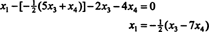Therefore, the set of solutions of the given homogeneous system can be written as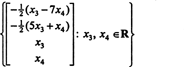which is a subspace of R 4. This is the nullspace of the matrixExample 3: Find the nullspace of the matrixBy definition, the nullspace of A consists of all vectors x such that A x = 0. Perform the following elementary row operations on A,to conclude that A x = 0 is equivalent to the simpler system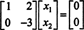The second row implies that x 2 = 0, and back‐substituting this into the first row implies that x 1 = 0 also. Since the only solution of A x = 0 is x = 0, the nullspace of A consists of the zero vector alone. This subspace, { 0}, is called the trivial subspace (of R 2).

Example 4: Find the nullspace of the matrix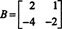To solve B x = 0, begin by row‐reducing B: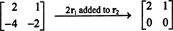The system B x = 0 is therefore equivalent to the simpler systemSince the bottom row of this coefficient matrix contains only zeros, x 2 can be taken as a free variable. The first row then gives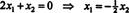so any vector of the form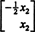satisfies B x = 0. The collection of all such vectors is the nullspace of B, a subspace of R 2: